# 程序员最近都爱上了这个网站  程序员们快来瞅瞅吧！  it98k网:it98k.com

出租广告位,需要合作请联系站长

+关注

## 1.1. Python的诞生

Python的作者是著名的“龟叔”Guido van Rossum，1989年，龟叔为了打发无聊的圣诞节，开始编写Python语言。1991年，第一个Python编译器诞生。它是用C语言实现的，并能够调用C语言的库文件。### 1.1.1. 提升自己的逼格，你需要了解

Python的发音与拼写
Python的意思是蟒蛇，是取自英国20世纪70年代首播的电视喜剧《蒙提.派森干的飞行马戏团》（Monty Python’s Flying Circus）
Python的作者是Guido van Rossum（龟叔）

Python是龟叔在1989年圣诞节期间，为了打发无聊的圣诞节而用C编写的一个编程语言

Python第一个公开发行版发行于1991年
Python目前有两个主要版本，Python2和Python3，最新版分别为2.7.12和3.7.2
Life is short, you need Python. 人生苦短，我用Python。1）官 网：https://www.python.org/ 2）中文社区：http://www.pythontab.com/

### 1.1.2. Python各个版本发布时间

1989年由荷兰人Guido van Rossum于1989年发明，第一个公开发行版发行于1991年

``````Python 1.0 - January 1994 增加了 lambda, map, filter and reduce.
Python 2.0 - October 16, 2000，加入了内存回收机制，构成了现在Python语言框架的基础
Python 2.4 - November 30, 2004, 同年目前最流行的WEB框架Django 诞生
Python 2.5 - September 19, 2006
Python 2.6 - October 1, 2008
Python 2.7 - July 3, 2010
Python 3.0 - December 3, 2008
Python 3.1 - June 27, 2009
Python 3.2 - February 20, 2011
Python 3.3 - September 29, 2012
Python 3.4 - March 16, 2014
Python 3.5 - September 13, 2015
``````

### 1.1.4. Python在一些公司的应用

CIA: 美国中情局网站就是用Python开发的
NASA: 美国航天局(NASA)大量使用Python进行数据分析和运算
Dropbox:美国最大的在线云存储网站，全部用Python实现，每天网站处理10亿个文件的上传和下载
Instagram:美国最大的图片分享社交网站，每天超过3千万张照片被分享，全部用python开发
Redhat: 世界上最流行的Linux发行版本中的yum包管理工具就是用python开发的

## 1.2. Python 的安装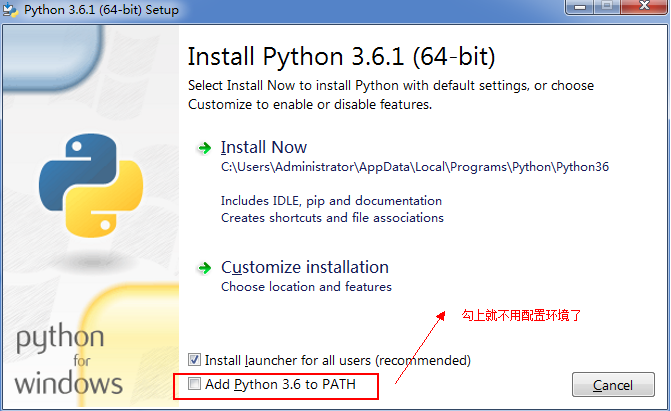Python安装.png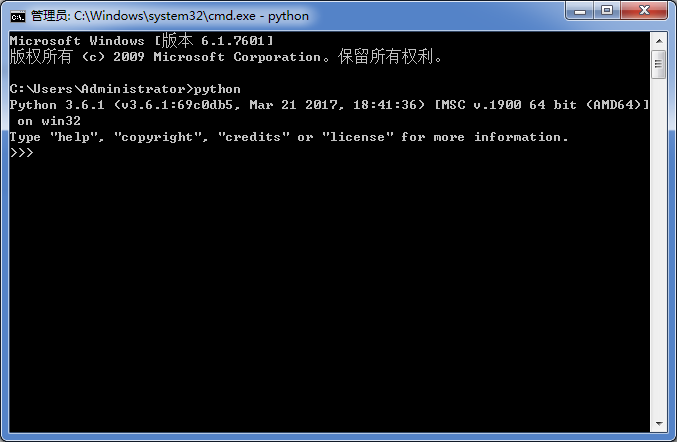## 1.3. Python中文文档

https://docs.python.org/zh-cn/3/

## 1.4. 集成开发环境 IDE

### 1.4.1. 在Pycharm上运行第一个Python应用程序

#### 1.4.2. 1新建项目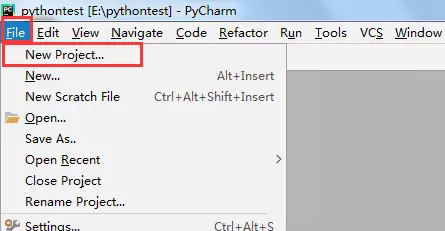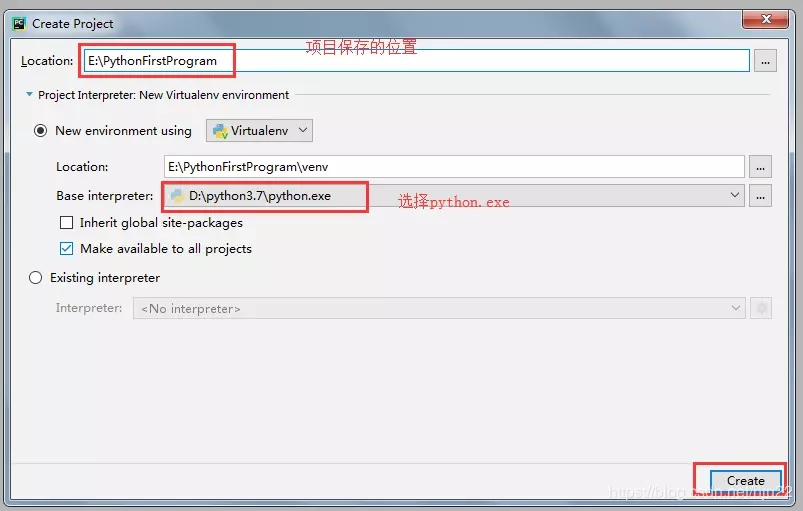3、弹出的窗口里点击ok，项目就创建好了#### 1.4.3. 2、新建python文件

1、右击项目，点击New ，再点击Python File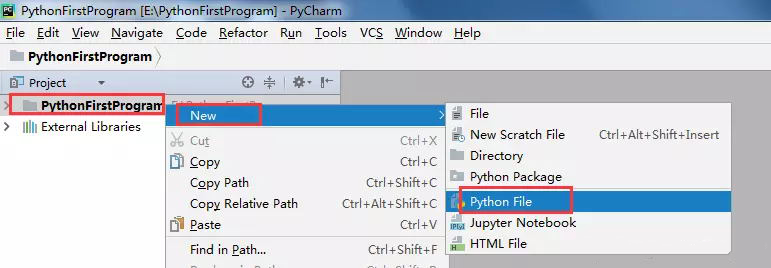2、输入要创建的python文件的名称，点击ok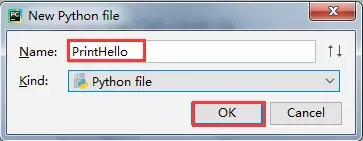#### 1.4.4. 3、写代码，运行

1、输入代码 print(“Hello world!”)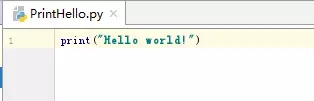image

2、右击空白处，选择Run ‘PrintHello’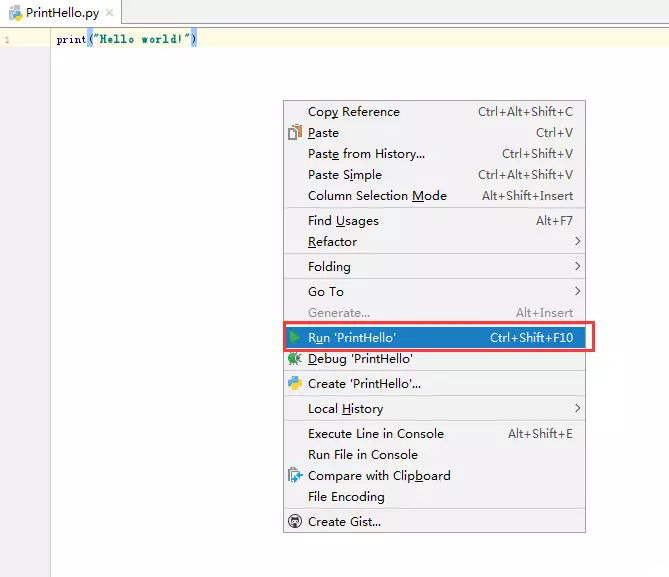#### 1.4.5. 4、运行结果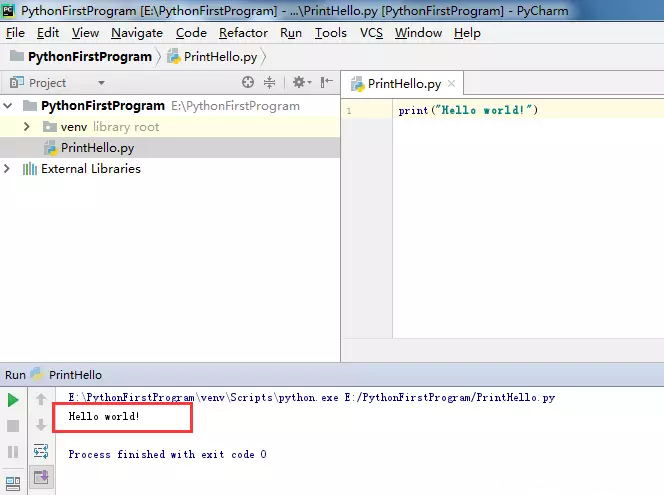## 2.1. 变量定义

= 左边是一个变量名
= 右边是存储在变量中的值

``````变量名 = 值
``````

### 2.1.1. 实例1—— 学生注册

``````# 定义变量---学生学号
studentNo = "9527"

# 定义变量---学生密码

# 在程序中，如果要输出变量的内容，需要使用 print 函数
print(studentNo)

``````

Python的代码缩进

Python语言通过缩进来组织代码块，这是Python的强制要求。 下面的代码有误：

``````# 定义变量---学生学号
studentNo = "9527"

# 定义变量---学生密码

# 在程序中，如果要输出变量的内容，需要使用 print 函数
print(studentNo)
``````

``````# 定义变量---学生学号
studentNo = "9527"

# 定义变量---学生密码

# 在程序中，如果要输出变量的内容，需要使用 print 函数
print(studentNo)
``````

### 2.1.2. 实例2—— 超市买菜

``````# 定义柿子价格变量
price = 8.5

# 定义购买重量
weight = 7.5

# 计算金额
money = price * weight

print(money)
``````

## 2.2. 变量的类型

### 2.2.1. 变量类型的演练 —— 表达个人信息

4 种
str —— 字符串
bool —— 布尔（真假）
int —— 整数
float —— 浮点数（小数）

Python 可以根据 = 等号右侧的值，自动推导出变量中存储数据的类型

### 2.2.2. 变量的类型

``````type(name)
``````

### 2.2.3. 不同类型变量之间的计算

1) 数字型变量 之间可以直接计算

True 对应的数字是 1
False 对应的数字是 0

``````i = 10
f = 10.5
b = True

print(i+f+b)
``````
``````21.5
``````

2) 字符串变量 之间使用 + 拼接字符串

``````first_name = "三"
last_name = "张"
print(first_name + last_name)
``````

3) 字符串变量 可以和 整数 使用 * 重复拼接相同的字符串

``````print("-" * 50)
``````

`````` '--------------------------------------------------'
``````

4) 数字型变量 和 字符串 之间 不能进行其他计算

``````first_name = "zhang"
x = 10

print( x + first_name)
---------------------------------------------------------------------------
TypeError: unsupported operand type(s) for +: 'int' and 'str'

``````

``````first_name = "zhang"
x = 10
print(str(x)+first_name)
``````

### 2.2.4. 变量的输入

1) 关于函数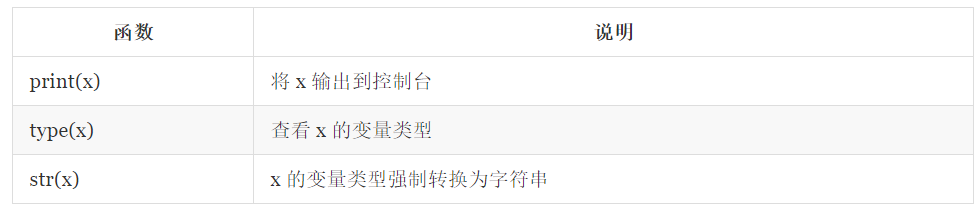2) input 函数实现键盘输入

``````字符串变量 = input("提示信息：")
``````

3) 其他类型转换函数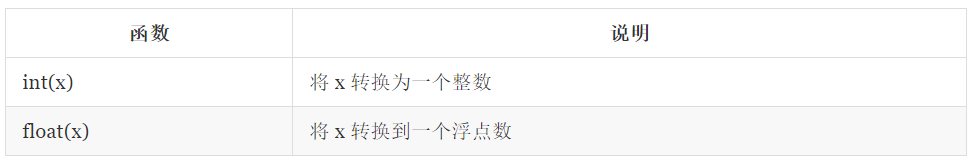4) 变量输入演练 —— 超市买苹果

``````# 1. 输入苹果单价
price_str = input("请输入苹果价格：")

# 2. 要求苹果重量
weight_str = input("请输入苹果重量：")

# 3. 计算金额
# 1> 将苹果单价转换成小数
price = float(price_str)

# 2> 将苹果重量转换成小数
weight = float(weight_str)

# 3> 计算付款金额
money = price * weight

print(money)
``````

### 2.2.5. 变量的格式化输出

``````苹果单价 9.00 元／斤，购买了 5.00 斤，需要支付 45.00 元
``````

% 被称为 格式化操作符，专门用于处理字符串中的格式

% 和不同的 字符 连用，不同类型的数据 需要使用 不同的格式化字符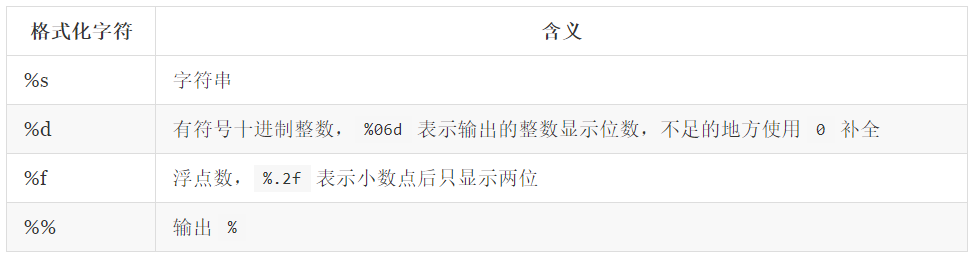``````print("格式化字符串" % 变量1)

print("格式化字符串" % (变量1, 变量2...))
``````

``````定义字符串变量 name，输出 我的名字叫 小明，请多多关照！

``````
``````print("我的名字叫 %s，请多多关照！" % name)
print("我的学号是 %06d" % student_no)
print("苹果单价 %.02f 元／斤，购买 %.02f 斤，需要支付 %.02f 元" % (price, weight, money))
print("数据比例是 %.02f%%" % (scale * 100))
``````

<模板字符串>.format(<逗号分隔的参数>)

``````str = "{}曰：学而时习之，不亦说乎。".format("孔子")
print(str)
``````
``````'孔子曰：学而时习之，不亦说乎。'
``````

``````"{}曰：学而时习之，不亦{}。".format("孔子","说乎")
``````
``````'孔子曰：学而时习之，不亦说乎。'
``````

``````"{1}曰：学而时习之，不亦{0}。".format("说乎","孔子")
``````
``````'孔子曰：学而时习之，不亦说乎。'
``````

f-字符串
f-string是 Python 3.6 中定义的一种参数化字符串的方式，主要是让格式化字符串更加便捷。 在 f-string 中，不要再使用 %-格式化 或者 format 函数中的复杂写法，可以直接在字符串中写入变量名。f-string 用 {} 标识变量，区别在于 {} 不再是用来占位的，而是直接写入变量名。 修改上面的格式化例子：

``````name = input('请输入姓名：')
fondness = input('请输入爱好：')
print(f'{name} 的爱好是 {fondness}！')    # 字符串前面的 f 大小写均可
``````

``````**************************************************

**************************************************
``````
``````"""

"""
name = input("请输入姓名：")
company = input("请输入公司：")
title = input("请输入职位：")
phone = input("请输入电话：")
email = input("请输入邮箱：")

print("*" * 50)
print(company)
print()
print("%s (%s)" % (name, title))
print()
print("电话：%s" % phone)
print("邮箱：%s" % email)
print("*" * 50)
``````

## 2.3. 变量的命名

### 2.3.1. 标识符和关键字

#### 1.1 标识符

``````c1 = '小朱学长'
n = 1500
``````

``````company = '小朱学长'
employeeNum = 1500
``````

#### 1.2 关键字

``````import keyword
print(keyword.kwlist)
``````
``````['False', 'None', 'True', 'and', 'as', 'assert', 'break', 'class', 'continue', 'def', 'del', 'elif', 'else', 'except', 'finally',
'for', 'from', 'global', 'if', 'import', 'in', 'is', 'lambda', 'nonlocal', 'not', 'or', 'pass', 'raise', 'return', 'try', 'while',
'with', 'yield']
``````

import 关键字 可以导入一个 “工具包”

### 2.3.2. 变量的命名规则

``````userName = 'zhangsan'
``````
``````Traceback (most recent call last):
File "C:/Users/Administrator/PycharmProjects/hellopython/test.py", line 2, in <module>
NameError: name 'username' is not defined
``````

## 2.4驼峰命名法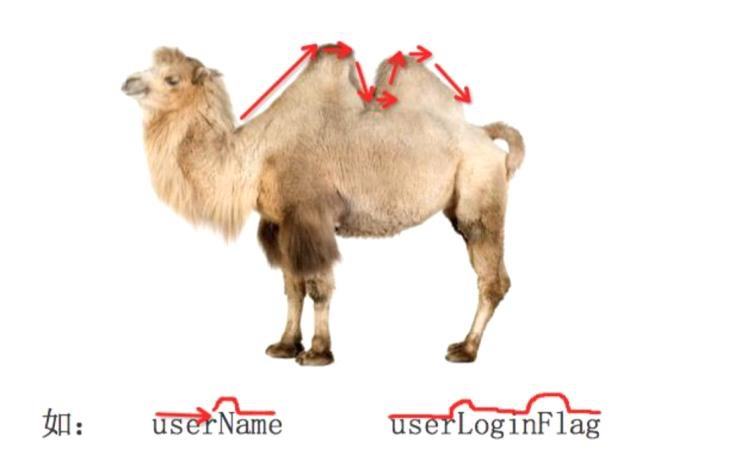## 3.1运算符

### 3.1.1. 算数运算符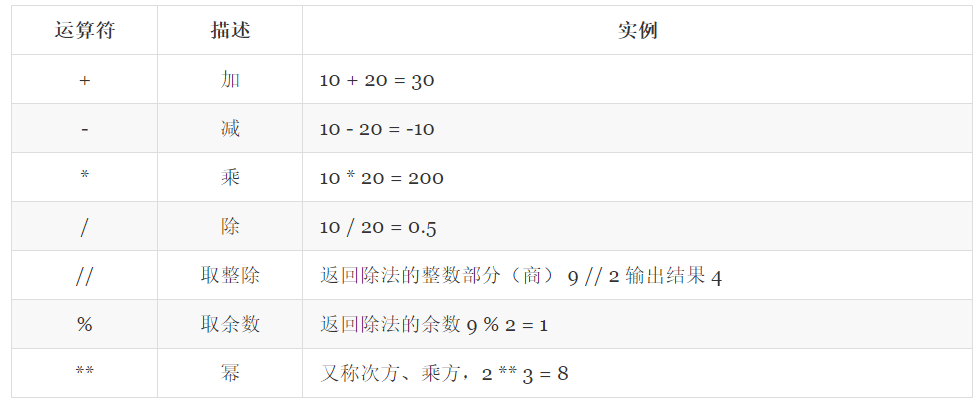``````>>> a=10
>>> b=5
>>> print(a+b)
15
>>> print(a-b)
5
>>> print(a*b)
50
>>> print(a/b)
2.0
>>> print(a**b)
100000
>>> print(a//b)
2
>>> print(a%b)
0
``````

### 3.1.2. 比较（关系）运算符``````>>> a=10
>>> b=20
>>> a==b
False
>>> a!=b
True
>>> a>b
False
>>> a<b
True
>>> a>=b
False
>>> a<=b
True
``````

### 3.1.3. 逻辑运算符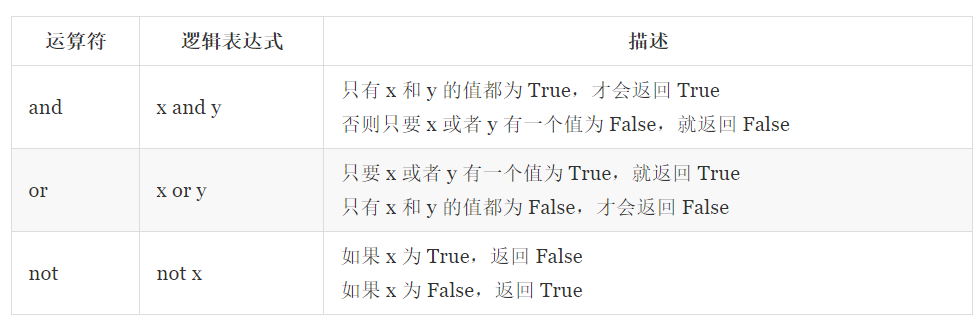``````>>> a=True
>>> b=False
>>> a and b
False
>>> a or b
True
>>> not a
False
>>> not -1
False
>>> not 0
True
``````

### 3.1.4. 赋值运算符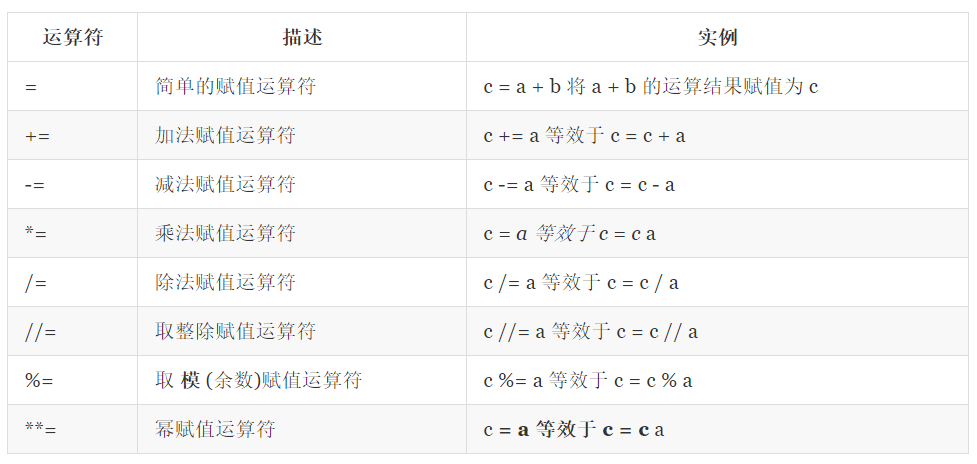``````>>> a=10
>>> b=20
>>> c=0
>>> c=a+b
>>> print(c)
30
>>> c+=10
>>> print(c)
40
>>> c-=a
>>> print(c)
30
>>> c*=a
>>> print(c)
300
>>> c/=a
>>> print(c)
30.0
>>> c%=a
>>> print(c)
0.0
>>> c=a**5
>>> print(c)
100000
>>> c//=b
>>> print(c)
5000
>>> print(b)
20
``````

### 3.1.5. 运算符的优先级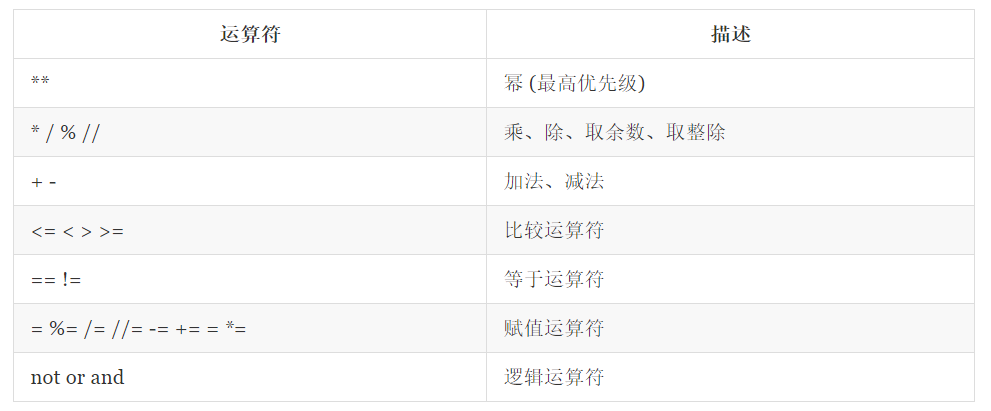## 4.1. 条件语句

Python 条件语句跟其他语言基本一致的，都是通过一条或多条语句的执行结果（ True 或者 False ）来决定执行的代码块。

Python 程序语言指定任何非 0 和非空（None）值为 True，0 或者 None为 False。

### 4.1.1. if 语句的基本形式

Python 中，if 语句的基本形式如下：

``````if 判断条件：
执行语句……
else：
执行语句……
``````

if 语句的判断条件可以用>（大于）、<(小于)、==（等于）、>=（大于等于）、<=（小于等于）来表示其关系。

``````# -*-coding:utf-8-*-

results=59

if results>=60:
print ('及格')
else :
print ('不及格')
``````

``````不及格
``````

``````num = 6
if num :
print('Hello Python')
``````

### 4.1.2. if 语句多个判断条件的形式

``````if 判断条件1:
执行语句1……
elif 判断条件2:
执行语句2……
elif 判断条件3:
执行语句3……
else:
执行语句4……
``````

``````# -*-coding:utf-8-*-

results = 89

if results > 90:
print('优秀')
elif results > 80:
print('良好')
elif results > 60:
print ('及格')
else :
print ('不及格')
``````

``````良好
``````

### 4.1.3. if 语句多个条件同时判断

Python 不像 Java 有 switch 语句，所以多个条件判断，只能用 elif 来实现，但是有时候需要多个条件需同时判断时，可以使用 or （或），表示两个条件有一个成立时判断条件成功；使用 and （与）时，表示只有两个条件同时成立的情况下，判断条件才成功。

``````# -*-coding:utf-8-*-

java = 86
python = 68

if java > 80 and  python > 80:
print('优秀')
else :
print('不优秀')
``````

``````不优秀
``````

``````java = 86
python = 68

if (80 <= java < 90) or (80 <= python < 90):
print('良好')
``````

``````良好
``````

``````我想买车，买什么车决定于我在银行有多少存款

``````

``````输入小明的考试成绩，显示所获奖励

90分>成绩>=60分，妈妈给他买本参考书

``````

## 4.2. 循环语句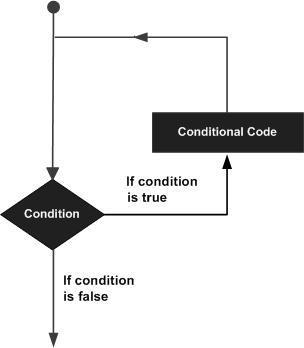Python 提供了 for 循环和 while 循环，当然还有一些控制循环的语句：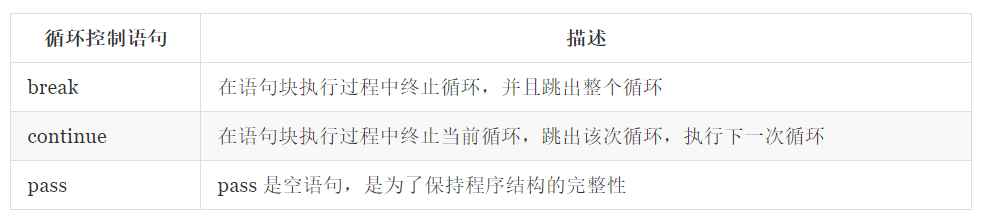### 4.2.1. While 循环语句

``````count = 1
sum = 0
while count <= 100:
sum = sum + count
count = count + 1
print(sum)
``````

``````5050
``````

``````count = 1
sum = 0
while  count <= 100:
sum = sum + count
if  sum > 1000:  #当 sum 大于 1000 的时候退出循环
break
count = count + 1
print(sum)
``````

``````1035
``````

``````count = 1
sum = 0
while  count <= 100:
if count % 2 == 0:  # 双数时跳过输出
count = count + 1
continue
sum = sum + count
count = count + 1
print(sum)
``````

``````2500
``````

``````count = 0
while count < 5:
print (count)
count = count + 1
else:
print (count)
``````

``````0
1
2
3
4
5
``````

### 4.2.2. for 循环语句

for循环可以遍历任何序列的项目，如一个字符串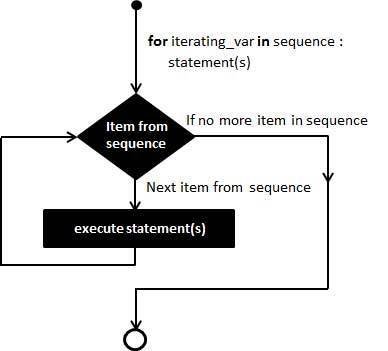``````for iterating_var in sequence:
statements(s)
``````

``````for letter in 'www.neuedu.com':
print(letter)
``````

``````w
w
w
.
n
e
u
e
d
u
.
c
o
m
``````
``````range()函数
``````

Python函数range()让你能够轻松地生成一系列的数字。例如，可以像下面这样使用函数range()来打印一系列的数字：

``````for value in range(1,5):
print(value)
``````

``````1
2
3
4
``````

``````for value in range(1,6):
print(value)
``````

``````    1
2
3
4
5
``````

### 4.2.3. 嵌套循环

Python 语言允许在一个循环体里面嵌入另一个循环。上面的实例也是使用了嵌套循环的。

for 循环嵌套语法

``````for iterating_var in sequence:
for iterating_var in sequence:
statements(s)
statements(s)
``````

while 循环嵌套语法

``````while expression:
while expression:
statement(s)
statement(s)
``````

``````for num in range(10,20):  # 迭代 10 到 20 之间的数字
for i in range(2,num): # 根据因子迭代
if num%i == 0:      # 确定第一个因子
j=num/i          # 计算第二个因子
print ('%d 是一个合数' % num)
break            # 跳出当前循环
else:                  # 循环的 else 部分
print ('%d 是一个质数' % num)
``````

``````10 是一个合数
11 是一个质数
12 是一个合数
13 是一个质数
14 是一个合数
15 是一个合数
16 是一个合数
17 是一个质数
18 是一个合数
19 是一个质数
``````

``````# 打印九九乘法表
for i in range(1, 10):
for j in range(1, i+1):
print('{}x{}={}\t'.format(i, j, i*j), end='')
print()
``````
``````1x1=1
2x1=2   2x2=4
3x1=3   3x2=6   3x3=9
4x1=4   4x2=8   4x3=12  4x4=16
5x1=5   5x2=10  5x3=15  5x4=20  5x5=25
6x1=6   6x2=12  6x3=18  6x4=24  6x5=30  6x6=36
7x1=7   7x2=14  7x3=21  7x4=28  7x5=35  7x6=42  7x7=49
8x1=8   8x2=16  8x3=24  8x4=32  8x5=40  8x6=48  8x7=56  8x8=64
9x1=9   9x2=18  9x3=27  9x4=36  9x5=45  9x6=54  9x7=63  9x8=72  9x9=81
``````

## 4.3. 随机数的处理

``````import random
``````

random.randint(a, b) ，返回 [a, b] 之间的整数，包含 a 和 b

``````random.randint(12, 20)  # 生成的随机数n: 12 <= n <= 20
random.randint(20, 20)  # 结果永远是 20
random.randint(20, 10)  # 该语句是错误的，下限必须小于上限
``````

``````Enter the smaller number: 1
Enter the larger number: 20
Too small
Too small
Too small
Too large
You've got it in 5 tries!
``````
``````import random

smaller = int(input("Enter the smaller number: "))
larger = int(input("Enter the larger number: "))
myNumber = random.randint(smaller, larger)
count = 0
while True:
count += 1
userNumber = int(input("Enter your guess: "))
if userNumber < myNumber:
print("Too small")
elif userNumber > myNumber:
print("Too large")
else:
print("You've got it in", count, "tries!")
break
``````

## 5.1. 列表定义

``````[1,2,3,2.4,5]
[0.01,"zhangsan",[1,2,3]]
``````

``````>>>x =[1,2,3]

>>>del  x                    #删除指定位置元素

>>>x

[1，3]

>>>del x                        #删除整个列表

>>>x

Traceback (most recent call last):

File "<pyshell#6>", line 1, in <module>

x

NameError: name 'x' is not defined
``````

## 5.2. 列表元素的增加

### 5.2.1. append()

``````>>>x = [1,2,3]

>>>x.append(4)

>>>x

[1,2,3,4]
``````

### 5.2.2. insert()

``````>>>x = [1,3,4]
>>>x.insert(1,2)
>>>x
[1,2,3,4]
``````

### 5.2.3. extend()

``````>>>x = [1,2]

>>>x.extend([3,4])

>>>x

[1,2,3,4]
``````

## 5.3. 运算符" + " 和" * "

``````>>> x = [1,2,3]
>>>y = x + 
>>>y
[1,2,3,4]
>>>y = x*2
>>>y
[1,2,3,4,1,2,3,4]
``````

## 5.4. 列表元素的删除

### 5.4.1. pop()

``````>>>x = [1,2,3,4]
>>>x.pop()                        #弹出并返回尾部元素
4
>>>x.pop(1)                     #弹出并返回指定位置的元素
>>>2
``````

### 5.4.2. remove()

``````>>>x = [1,2,2,3,4]

>>>x.remove(2)

>>>x

[1,2,3,4]
``````

### 5.4.3. clear()

``````>>>x = [1,2,3,4]

>>>x.clear()

>>>x

[ ]
``````

### 5.4.4. del

``````>>>x = [1,2,3,4]

>>>del x

>>>x

[1,3,4]
``````

## 5.5. 列表元素访问与计数

### 5.5.1. count()

``````>>> x =[1,2,3,3,4,5]

>>>x.count(3)

2

>>>x.count(0)

0
``````

### 1.5.2. index()

``````>>>x = [1,2,3,2,2,4]

>>>x.index(2)

1
``````

### 5.5.3. in

``````>>>x = [1,2,3,4]

>>>3 in x

True

>>>5 in x

False
``````

## 5.6. 列表排序

### 5.6.1. sort()

``````>>> aList = [3, 4, 5, 6, 7, 9, 11, 13, 15, 17]

>>> import random

>>> random.shuffle(aList)                              #随机降序

>>> aList

[3, 4, 15, 11, 9, 17, 13, 6, 7, 5]

>>> aList.sort()                                              #默认是升序排序

>>> aList.sort(reverse = True)                       #降序排序

>>> aList

[17, 15, 13, 11, 9, 7, 6, 5, 4, 3]
``````

### 5.6.2. reverse()

``````>>>x = [1,3,2,4,6,5]

>>>x.reverse()

>>>x

[5,6,4,2,3,1]
``````

### 5.6.3. sorted()

``````>>>x = [6,4,2,4,1]

>>> sorted(x)                                     #升序排序

[1,2,4,4,6]

>>> sorted(aList,reverse = True)             #降序排序

[6,4,4,2,1]

>>>x

[6,4,2,4,1]
``````

### 5.6.4. reversed()

``````>>>x =[1,5,3,6,2]

>>>list(reversed(x))

[2,6,3,5,1]

>>>x

[1,5,3,6,2]
``````

## 5.7. 用于序列操作的常用内置函数

### 5.7.4. zip()

``````>>> aList = [1, 2, 3]

>>> bList = [4, 5, 6]

>>> cList = zip(a, b)                               #返回zip对象

>>> cList

<zip object at 0x0000000003728908>

>>> list(cList)                                         #把zip对象转换成列表

[(1, 4), (2, 5), (3, 6)]
``````

### 5.7.5. enumerate()

``````>>> for item in enumerate('abcdef'):
print(item)
(0, 'a')

(1, 'b')

(2, 'c')

(3, 'd')

(4, 'e')

(5, 'f')
``````

## 5.8. 遍历列表的三种方式

``````a = ['a','b','c','d','e','f']
for i in a:
print(i)

for i in range(len(a)):
print(i,a[i])
for i,ele in enumerate(a):
print(i,ele)
``````

## 5.9. 列表推导式

``````lis = [i for i in range(100)]
print(lis)
``````

``````>>> vec = [[1,2,3], [4,5,6], [7,8,9]]

>>> [num for elem in vec for num in elem]

[1, 2, 3, 4, 5, 6, 7, 8, 9]
``````

``````>>> vec = [[1, 2, 3], [4, 5, 6], [7, 8, 9]]

>>> result = []

>>> for elem in vec:

for num in elem:

result.append(num)

>>> result

[1, 2, 3, 4, 5, 6, 7, 8, 9]
``````

``````>>> aList = [-1,-4,6,7.5,-2.3,9,-11]

>>> [i for i in aList if i>0]

[6, 7.5, 9]
``````

``````>>> [(x, y) for x in range(3) for y in range(3)]

[(0, 0), (0, 1), (0, 2), (1, 0), (1, 1), (1, 2), (2, 0), (2, 1), (2, 2)]

>>> [(x, y) for x in [1, 2, 3] for y in [3, 1, 4] if x != y]

[(1, 3), (1, 4), (2, 3), (2, 1), (2, 4), (3, 1), (3, 4)]
``````

``````products = [['iphone',6888],['三星',3000],['小米',2500]]
shopping_car = []
flag = True
while flag:
print("******商品列表******")
for index,i in enumerate(products):
print("%s.  %s|  %s" %(index,i,i))
choice = input("请输入您想购买的商品的编号：")
if choice.isdigit():#isdigit()判断变量是什么类型
choice = int(choice)
if choice>=0 and choice<len(products):
shopping_car.append(products[choice])
print("已经将%s加入购物车" %(products[choice]))
else:
print("该商品不存在")
elif choice == "q":
if len(shopping_car)>0:
print("您打算购买以下商品：")
for index,i in enumerate(shopping_car):
print("%s. %s|  %s" %(index,i,i))
else:
print("您的购物车中没有添加商品")
flag = False
``````

## 5.10. 列表切片

``````  colors=["red","blue","green"]

colors =="red"

colors=="blue"
``````

`````` colors[-1]=="green"
``````

### 5.10.1. 列表的切片操作

``````  li=["A","B","C","D"]
``````

start是切片起点索引，end是切片终点索引，但切片结果不包括终点索引的值。step是步长默认是1。

``````     t=li[0:3]        ["A","B","C"]        #起点的0索引可以省略，t=li[:3]

t=li[2: ]        ["C","D"]           #省略end，则切到末尾

t=li[1:3]        ["B","C"]

t=li[0:4:2]       ["A","C"]       #从li到li,设定步长为2。
``````

``````            t=li[0:2]

t=li[0:-2]

t=li[-4:-2]

t=li[-4:2]
``````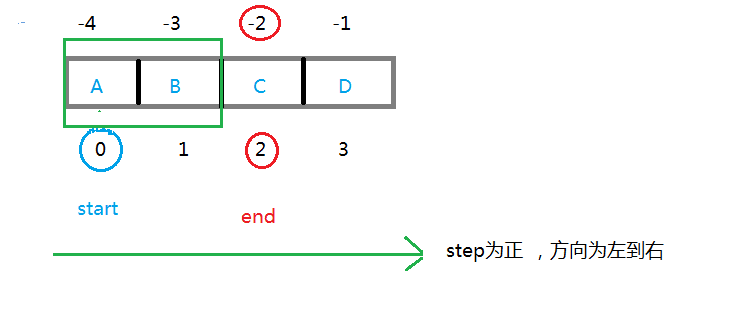``````         t=li[-1:-3:-1]

t=li[-1:1:-1]

t=li[3:1:-1]

t=li[3:-3:-1]
``````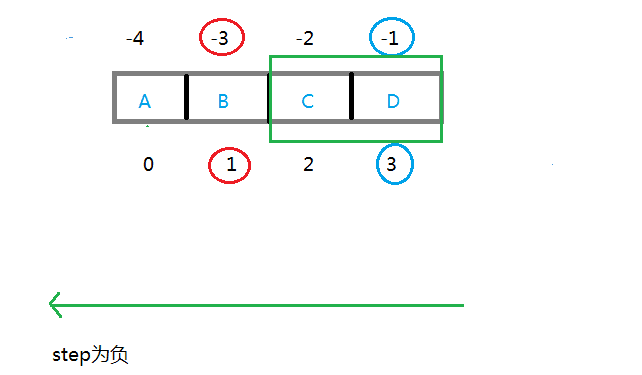``````         t=li[-1:-3]

t=li[-1:1]

t=li[3:1]

t=li[3:-3]
``````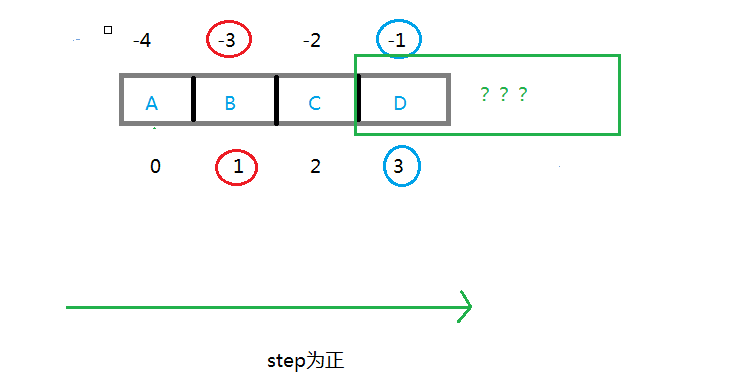``````t=li[::-1]     t--->["C","B","A"]     #反向切，切出全部

t=li[:]        t--->["A","B","C","D"]   #正向切全部
``````

### 5.10.2. 使用切片来原地修改列表内容

``````>>> aList = [3, 5, 7]

>>> aList[len(aList):] =                #在尾部追加元素

>>> aList

[3, 5, 7, 9]

>>> aList[:3] = [1, 2, 3]                  #替换前3个元素

>>> aList

[1, 2, 3, 9]

>>> aList[:3] = []                         #删除前3个元素

>>> aList


``````

### 5.10.3. 使用del与切片结合来删除列表元素

``````>>> aList = [3,5,7,9,11]

>>> del aList[:3]                          #删除前3个元素

>>> aList

[9, 11]

>>> aList = [3,5,7,9,11]

>>> del aList[::2]                         #删除偶数位置上的元素

>>> aList

[5, 9]
``````

### 5.10.4. 切片返回的是列表元素的浅复制

``````>>> aList = [3, 5, 7]

>>> bList = aList              #bList与aList指向同一个内存

>>> bList

[3, 5, 7]

>>> bList = 8                #修改其中一个对象会影响另一个

>>> aList

[3, 8, 7]

>>> aList == bList             #两个列表的元素完全一样

True

>>> aList is bList              #两个列表是同一个对象

True

>>> id(aList)                     #内存地址相同

19061816

>>> id(bList)

19061816

>>> aList = [3, 5, 7]

>>> bList = aList[::]                 #切片，浅复制

>>> aList == bList                    #两个列表的元素完全一样

True

>>> aList is bList                    #但不是同一个对象

False

>>> id(aList) == id(bList)            #内存地址不一样

False

>>> bList = 8                      #修改其中一个不会影响另一个

>>> bList

[3, 8, 7]

>>> aList

[3, 5, 7]
``````

## 5.11. 元组

### 5.11.1. 元组的创建与删除

``````>>>x = (1,2,3)                               #直接把元组赋值给一个变量
>>>x
(1,2,3)
``````

``````x =(3)
print(type(x))
x =(3,)
print(type(x))
``````

``````<class 'int'>
<class 'tuple'>
``````

``````>>>x = ()                                       #空元组

>>>x

()

>>>x = tuple()                              #空元组

>>>x

()
``````

``````>>>tuple(range(5))

(0,1,2,3,4)

>>> tuple('abcdefg')                          #把字符串转换为元组

('a', 'b', 'c', 'd', 'e', 'f', 'g')

>>> aList

[-1, -4, 6, 7.5, -2.3, 9, -11]

>>> tuple(aList)                                #把列表转换为元组

(-1, -4, 6, 7.5, -2.3, 9, -11)
``````

### 5.11.2. 元组与列表的区别

1.元组中的数据一旦定义就不允许更改。
2.元组没有append()、extend()和insert()等方法，无法向元组中添加元素。
3.元组没有remove()或pop()方法，也无法对元组元素进行del操作，不能从元组中删除元素。
4.从效果上看，tuple( )冻结列表，而list( )融化元组。

## 5.12. 元组的优点

1.元组的速度比列表更快。
2.元组对不需要改变的数据进行“写保护”将使得代码更加安全。
3.元组可用作字典键（特别是包含字符串、数值和其它元组这样的不可变数据的元组）。元组有时还作为函数的返回值返回（返回多个值）。

## 6.1. 字符串的常用操作方法

### 6.1.1. 字符串切片

``````lang = '小朱学长'
print(lang)
print(lang)
``````
``````小

``````

``````lang[2:4]
# 学长
``````

``````lang[2:]
# 学长
``````

``````lang[:3]
# 小朱学
``````

``````    lang[:]
# 小朱学长
``````

``````    lang[3:100]
# 长

``````

``````    lang[-2:]
# 学长
lang[-2,2]
# ''
``````

``````s = 'www.neuedu.com'
#### 切片取出来的字符串与原字符串无关，原字符串不变
print(s[6: 10])
print(s[7:: 2])
``````
``````uedu
eucm
``````

``````print(s[-1: -4: -1])
print(s[-1: 2]) #### 取不到数据，因为步长默认-1
print(s[-1: 2: -1])#### 可以
``````
``````moc

moc.udeuen.
``````

### 6.1.2. 把字符串全部大写或小写upper， lower

``````s = 'www.NEUEDU.com'
print(s.upper())    #### 全部大写
print(s.lower())    #### 全部小写
``````

### 6.1.3. 判读以xx开头或结尾startswith，endswith

``````s = 'www.neuedu.com'
print(s.startswith('www'))    #### 判断是否以www开头
print(s.endswith('com'))    #### 判断是否以com结尾
``````
``````True
True
``````

### 6.1.4. 查找元素find ，index

``````s = 'chhengt'
print(s.find('h'))  #### 通过元素找索引找到第一个就返回（可切片）
print(s.find('b'))  #### 找不到返回 -1
print(s.index('b'))   #### 找不到会报错
``````
``````Traceback (most recent call last):
1
File "C:/Users/Administrator/PycharmProjects/textgame/tests/test_bag.py", line 4, in <module>
-1
print(s.index('b'))   #### 找不到会报错
``````

### 6.1.5. strip 默认去除字符前后两端的空格， 换行符， tab

``````s = 'qqwalex qqwusir barryy'
print(s.strip('qqw'))
print(s.strip(''))
print(s.lstrip('yy'))
print(s.rstrip('yy'))
``````
``````alex qqwusir barryy
qqwalex qqwusir barryy
qqwalex qqwusir barryy
qqwalex qqwusir barr
``````

### 6.1.6. split 把字符串分割成列表

``````#### 分割出的元素比分隔符数+1  ***
#### 字符串变成->>>列表
s = 'qqwalex qqwusir barryy'
s1 = 'qqwale;x qqwu;sir bar;ryy'
print(s.split())    #### 默认以空格分割
print(s1.split(';'))    #### 以指定字符分割
print(s1.split(';', 1)) #### 指定分割多少个
``````
``````['qqwalex', 'qqwusir', 'barryy']
['qqwale', 'x qqwu', 'sir bar', 'ryy']
['qqwale', 'x qqwu', 'sir bar;ryy']
``````

### 6.1.7. join把列表转化成字符串

``````#### 列表转化成字符串 list --> str
s = 'alex'####看成字符列表
li = ['aa', 'ddj', 'kk']    #### 必须全是字符串
s1 = '_'.join(s)
print(s1)
s2 = ' '.join(li)
print(s2)
``````
``````a_l_e_x
aa ddj kk
``````

### 6.1.8. is系列

``````#### 字符串.isalnum()    所有字符都是数字或者字母，为真返回 Ture，否则返回 False。
#### 字符串.isalpha()     所有字符都是字母，为真返回 Ture，否则返回 False。
#### 字符串.isdigit()     所有字符都是数字，为真返回 Ture，否则返回 False。
#### 字符串.islower()    所有字符都是小写，为真返回 Ture，否则返回 False。
#### 字符串.isupper()    所有字符都是大写，为真返回 Ture，否则返回 False。
#### 字符串.istitle()            所有单词都是首字母大写，为真返回 Ture，否则返回 False。
#### 字符串.isspace()    所有字符都是空白字符，为真返回 Ture，否则返回 False。

#### is 系列
name = 'taibai123'
print(name.isalnum())   #### 字符串由数字或字母组成时返回真
print(name.isalpha())   #### 字符只由字母组成时返回真
print(name.isdigit())   #### 字符只由数字组成时返回真
``````

### 6.1.9. count 计算字符串中某个字符出现的次数 *

``````#### count 计算字符串中某个字符出现的次数  ***
s = 'www.neuedu.com'
print(s.count('u'))
print(s.count('u', 7))
``````
``````2
1
``````

### 6.1.10. replace 替换字符串中指定的字符

``````s = 'asdf 之一，asdf也，asdf'
#### replace  ***
s1 = s.replace('asdf', '日天')
print(s1)
s1 = s.replace('asdf', '日天', 1)
print(s1)
``````
``````日天 之一，日天也，日天

``````

### 6.1.11. format格式化输出

``````#### format 格式化输出  ***
#### 第一种方式：
s = '我叫{}, 今年{}， 性别{}'.format('小五', 25, '女')
print(s)
#### 第二种方式
s1 = '我叫{0}, 今年{1}， 性别{2}，我依然叫{0}'.format('小五', 25, '女')
print(s1)
#### 第三种方式
s2 = '我叫{name}, 今年{age}， 性别{sex}，我依然叫{name}'.format(age=25, sex='女',name='小五')
print(s2)
``````
``````我叫小五, 今年25， 性别女

``````

### 6.1.12. capitalize() 首字母大写

``````s = 'chen wuang4fhsa￥fh。f'
#### capitalize() 首字母大写 **
s1 = s.capitalize()
print(s1)
``````
``````Chen wuang4fhsa￥fh。f
``````

### 6.1.13. center() 将字符串居中可以设置总长度，可以设置填充物

``````#### center() 将字符串居中可以设置总长度，可以设置填充物 *
s = 'chenziwu'
s2 = s.center(50)
s2 = s.center(50, '*')
print(s2)
``````
``````*********************chenziwu*********************
``````

### 6.1.14. title 非字母隔开的每个单词的首字母大写 *

``````s = 'chen wuang4fhsa￥fh。f'
#### title  非字母隔开的每个单词的首字母大写 *
s4 = s.title()
print(s4)
``````
``````Chen Wuang4Fhsa￥Fh。F
``````

### 6.1.15. 字符串是不可变变量，不支持直接通过下标修改

``````msg = 'abcdefg'
# msg = 'z'

msg = msg[:2] + 'z' + msg[3:]

print(msg)
``````

### 6.2. 综合练习 龟兔赛跑(模拟龟兔赛跑)本练习中要模拟龟兔赛跑的寓言故事。用随机数产生器建立模拟龟兔赛跑的程序。 对手从70个方格的第1格开始起跑，每格表示跑道上的一个可能位置，终点线在第70格处。 第一个到达终点的选手奖励一个新鲜萝卜和莴苣。兔子要在山坡上睡一觉，因此可能失去冠军。 有一个每秒钟滴答一次的钟，程序应按下列规则调整动物的位置：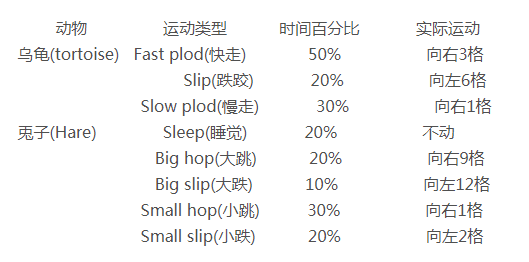``````from random import randint
import time

print('begin')
hPos = 0
tPos = 0

while True:
paodao = '_' * 70
n = randint(0,10) + 1
if n <= 5:
tPos += 3
elif 5 < n <= 7:
tPos -= 6
else:
tPos += 1

if n <= 2:
hPos = hPos
elif 3 <= n <= 4:
hPos += 9
elif n == 5:
hPos -= 12
elif 6 <= n <= 8:
hPos += 1
else:
hPos -= 2

if tPos >= 70 or hPos >= 70:
break

if tPos == hPos:
paodao = paodao[:tPos] + '咬' + paodao[tPos:]
else:
paodao = paodao[:tPos] + '龟' + paodao[tPos:]
paodao = paodao[:hPos] + '兔' + paodao[hPos:]

print(paodao)
time.sleep(0.5)

if tPos > 70:
print('龟赢')
else:
print('兔赢')
``````

## 6.3. 综合练习 猜单词游戏1.掌握字符串常用操作 2.掌握随机数的用法 3.掌握控制台基本输入输出 4.掌握循环，分支条件的用法 5.培养编程思维，提高分析问题能力`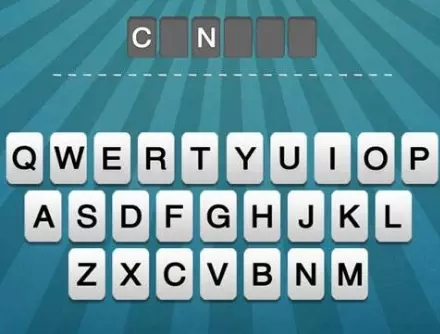``````import random
words = ['program','banana','tiger','policeman','interface']
index = random.randint(0,len(words) - 1)
word = words[index]
print(word)
wordbak = '-' * len(word)
print(wordbak)
guessTimes = 5
wordlst = list(wordbak)

while True:

if guessTimes <= 0:
break
if '-' not in wordlst:
break
char = input('请输入一个字符：')
if char in word:
for i,c in enumerate(word):
if c == char:
wordlst[i] = char
else:
guessTimes -= 1
print('你还剩下{}次机会'.format(guessTimes))

print(''.join(wordlst))

if guessTimes > 0:
print('you win')
else:
print('you lose')
``````

24 0

pdf(new) 更多>